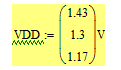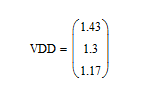cancel
Showing results for
Did you mean:
cancel
Showing results for
Did you mean:

Highlighted

## Accessing one mathcad script from another

Hi,

I need your help in solving the following problem -

Say, i have a main program worksheet ( Say A.xmcd ) where i have my inputs . Now my function ( set of calculations) is in another sheet ( Say B.xmcd )..

Now i need to pass the inputs from A.xcmd to B.xcmd and receive the ouput from B to A .

Say we have a very simple program in A.xcmd

input

x= 3

Function

f=cube (x)

B.xcmd

x1=x

value = x1*x1*x1

return(value)

The above stated are not exactly the syntax but a pseudo code .

How do i link A nd B from A ???

4 REPLIES 4
Highlighted

## Re: Accessing one mathcad script from another

You would have to use "Insert"/"Reference" to achieve what you want.

While it is possible that A references B and also B references A, I wouldn't recommend it. If you use just the function cube() in the attached sheet B, then there would be no need that B references A.

So I would suggest to avoid circular references and that you put your calculations written as functions (like cube()) in sheet B and reference it at the top of sheet A, which takes the input values and uses the functions written in sheet B.

See if the attached would help - it still uses the circular reference which works, because of the very simple mechanism: whenever you insert a reference is is as if you would type the whole content of the referenced file in that position. So see yourself, insert the reference to B in sheet A a second time and look what the value of x is. The function cube() will be defined two times that way which does no harm.

Highlighted

## Re: Accessing one mathcad script from another

Hey Werner ...

THanks..its working...

Highlighted

## Re: Accessing one mathcad script from another

Hello, I have a question regarding using "Reference" to link between Mathcad files.

I used this in a project, but my problem is that when I use the defined variable in the second sheet, if the variable defined has a unit measurement attached (such as Voltage - V), in the second sheet the unit measurement will not be attached:

Here is an example:

I defined the below variable VDD with unit "V" in x sheet.X sheet is referenced in Y sheet.

When I write VDD in Y sheet will appear this:without the unit...

Does anybody know how to fix this?

Highlighted

## Re: Accessing one mathcad script from another

I would suggest that you open a new thread for your question and also state which version of Mathcad (or probably Prime) you are using.

In this forum its unfortunately not possible to simply copy and paste pictures - you have to use the "Photos" icon to do so - unnecessarily awkward but thats the way this Lithium forum works.

From what I get from your description it looks like you have found a bug.

BTW - don't just send screenshots, attach the files, too!

EDIT: I just gave it a try with Mathcad 15 and even with Prime 5 and all worked OK as expected. The unit was not lost.